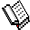Course Material Index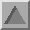Section Index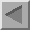Previous Page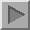Next Page

Bragg's Law

It is impossible to discuss powder diffraction much further without Bragg's Law. While its derivation will be considered in more detail later we need at least a basic understanding even at this stage.

Although Laue is credited with the discovery of diffraction, it fell to W.H.Bragg to devise an equation which predicted when diffraction would actually take place. This equation became known as Bragg's Law and must surely be one of the most well-known equations in science:

n λ = 2 d sin θ

where λ is the wavelength of the radiation used, d is the inter-planar spacing involved and θ is the angle between the incident (or diffracted) ray and the relevant crystal planes; n is an integer, referred to as the order of diffraction, and is often unity. These ideas are far from obvious for a newcomer to diffraction. An idea of its application may be gleaned from the following diagram depicting, schematically, X-ray diffraction from part of a single crystal: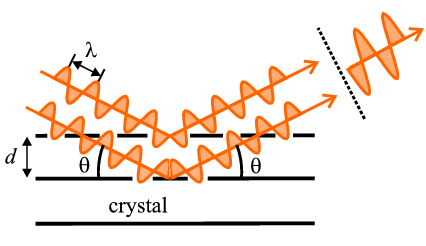The basic idea behind Bragg's Law is that, when it is satisfied, X-ray beams scattered from successive planes in the crystal (as shown) will travel distances differing by exactly one wavelength (for the case of n=1); this can be fairly easily proved from a geometrical consideration of the above diagram. In this precise direction, i.e. at the angle θ calculated by Bragg's Law, X-rays scattered from successive planes will interact constructively when they eventually reach the X-ray detector thus registering the passage of an intense beam which we call the diffracted beam. Of course it is not really the planes in the crystal that scatter the X-rays (since planes are abstract mathematical items) but rather the atoms with their electronic clouds. However this idea, developed by Bragg, of relating atoms to planes proved to be a great simplification in the subject and for this reason we still refer to individual diffracted beams as being "reflected" from certain "planes" even though we know it is the atoms that actually perform the scattering. At this point we do not need to understand the concept of planes in any greater depth than this, but for those who feel they would like to know a little more, you can click here.

One of the concluding ideas from Bragg's Law is that diffraction is, in effect, an "arranged event"; three parameters need to be harmonised: the wavelength of the X-rays, λ, the crystal orientation as defined by the angle, θ (see diagram above), and the spacing, d, of the crystal planes under consideration. For a given wavelength and set of planes one can conspire to arrange for diffraction to occur by, for example, continuously changing the orientation, i.e. changing theta, until a point arrives when Bragg's Law is satisfied: this is precisely when diffraction occurs.Course Material IndexSection IndexPrevious PageNext Page
 © Copyright 1997-2006.  Birkbeck College, University of London. Author(s): Paul Barnes Tony Csoka Simon Jacques